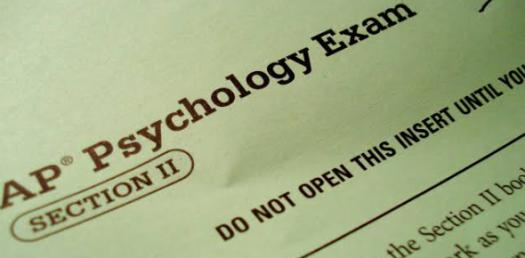# Advanced Placement: Quiz On Statistics! Trivia Questions

Approved & Edited by ProProfs Editorial Team
At ProProfs Quizzes, our dedicated in-house team of experts takes pride in their work. With a sharp eye for detail, they meticulously review each quiz. This ensures that every quiz, taken by over 100 million users, meets our standards of accuracy, clarity, and engagement.
| Written by Gian Petersen
G
Gian Petersen
Community Contributor
Quizzes Created: 14 | Total Attempts: 8,165
Questions: 5 | Attempts: 548SettingsWelcome to this advanced placement quiz on statistics quiz! The trivia questions are designed to help you test out what you have understood so far when it comes to your classes on the subject, and if you have been attentive. All the best as you tackle it and get to see just how much revision time you should get.

• 1.

### In a large high school, student leadership wants to randomly select a sample to survey the student body.  They will first randomly select 25 freshman, then randomly select 25 sophomores, then randomly select 25 juniors, and then randomly select 25 seniors.  This is an example of a

• A.

Cluster sample

• B.

Stratified sample

• C.

Simple random sample

• D.

Convenience sample

• E.

Systematic sample

B. Stratified sample
Explanation
The given scenario describes a stratified sample. In a stratified sample, the population is divided into distinct groups or strata, and a random sample is selected from each stratum. In this case, the student body is divided into four strata based on grade level (freshman, sophomore, junior, and senior), and a random sample of 25 students is selected from each stratum. This ensures that the sample represents the diversity of the student body across different grade levels.

Rate this question:

• 2.

### Below is a cumulative relative frequency plot (or, an ogive) for the scores of a large university class on a 90-point calculus exam.  Which of the following observations is correct?

• A.

The median score is at least 60 points.

• B.

The distribution of scores is skewed to the right.

• C.

The distribution of scores is skewed to the left.

• D.

The distribution of scores is roughly symmetric.

• E.

If a passing score is 60, most students passed the test.

B. The distribution of scores is skewed to the right.
Explanation
Based on the cumulative relative frequency plot, the correct answer is that the distribution of scores is skewed to the right. This can be determined by observing that the curve of the plot rises more steeply on the left side and gradually levels off on the right side, indicating that there are more lower scores and fewer higher scores. This is characteristic of a right-skewed distribution.

Rate this question:

• 3.

### A least-squares regression line for predicting performance on a college entrance exam based on high school GPA is determined to be Predicted Score = 273.5 + 91.2(GPA).  One student in the study had a high school GPA of 3.0, and an exam score of 510.  What is the residual for this student?

• A.

26.2

• B.

43.9

• C.

-37.1

• D.

-26.2

• E.

37.1

C. -37.1
Explanation
The residual for a student is the difference between the actual value and the predicted value. In this case, the predicted score for a student with a high school GPA of 3.0 would be 273.5 + 91.2(3.0) = 547.1. The actual score for this student is 510. Therefore, the residual is 510 - 547.1 = -37.1.

Rate this question:

• 4.

### A recent poll reported that 43% of Californians approve of the job the Governor is doing, with a margin of sampling error of + or - 3.25% at a 95% level of confidence.  Which of these correctly interprets that margin of error?

• A.

There is a 3.2% chance that the proportion of all Californians who approve of the job the Governor is doing is not 43%.

• B.

There is a 95% chance that the proportion of all Americans who approve of the job the Governor is doing is 43%.

• C.

About 3.2% of all Californians approve of 95% of what the Governor does.

• D.

About 95% of polls conducted this way will give a sample proportion within 3.2 percentage points of the actual proportion of all Californians who approve of the job the Governor is doing.

• E.

About 95% of polls conducted in this way will find between 39.8% and 46.2% of those sampled approve of the job the Governor is doing.

D. About 95% of polls conducted this way will give a sample proportion within 3.2 percentage points of the actual proportion of all Californians who approve of the job the Governor is doing.
Explanation
The correct answer correctly interprets the margin of error by stating that about 95% of polls conducted in this way will give a sample proportion within 3.2 percentage points of the actual proportion of all Californians who approve of the job the Governor is doing. This means that the reported approval rate of 43% could range from 39.8% to 46.2% in reality, with a high level of confidence.

Rate this question:

• 5.

### A test for heartworm in dogs shows a positive result in 96% of dogs that actually have heartworm, and shows negative results in 98% of dogs with no heartworm.  If heartworm actually occurs in 10% of dogs, what is the approximate probability that a randomly selected dog that tested positive for heartworm actually has heartworm?

• A.

11%

• B.

18%

• C.

84%

• D.

88%

• E.

96%Back to top